# How to Use Wildcards?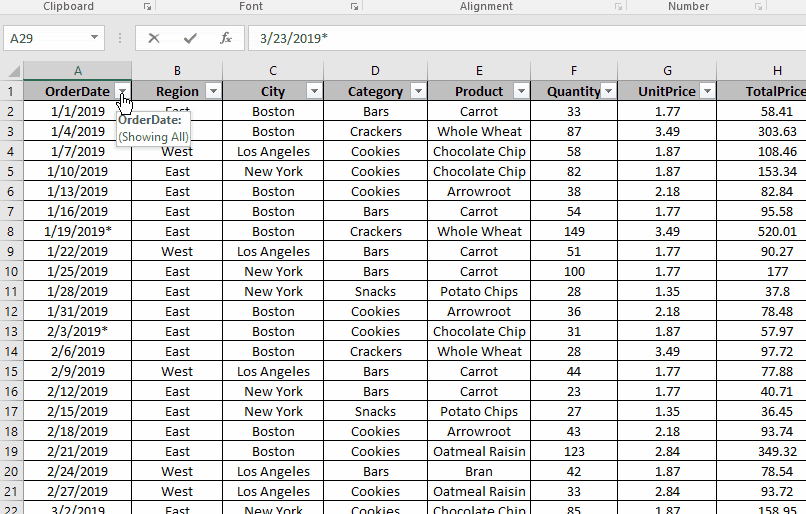In this article, we will learn about how and when to use Wildcard characters or in-built regex (regular expressions) characters for excel functions.

Excel lets you use Wildcard characters for catching strings and perform functions on it.

There are three wildcard characters in Excel

1. Question mark (?) : This wildcard is used to search for any single character.
2. Asterisk (*): This wildcard is used to find any number of characters preceding or following any character.
3. Tilde (~): This wildcard is an escape character, used preceding the question mark (?) or asterisk mark (*).

Let’s use these wildcards in examples

Here we need products which starts with C. So first we will apply filter to the list using Ctrl + Shift + L. Then in the Product search box type “C*” as shown below.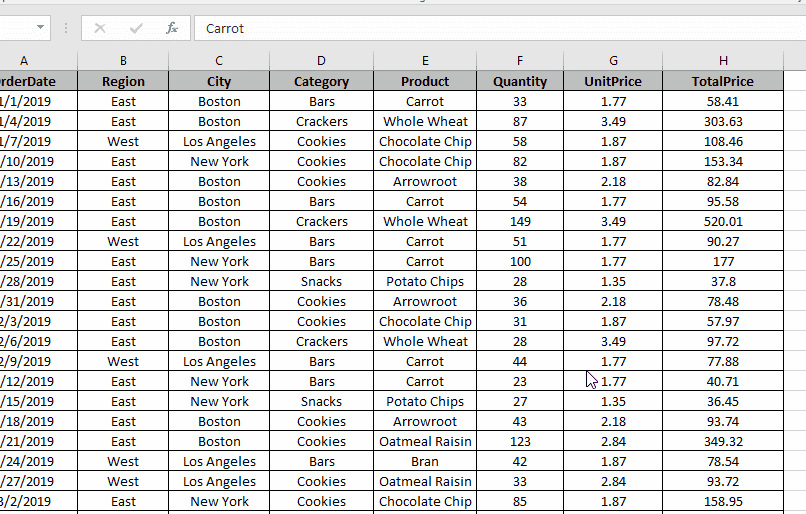As you can see the whole list is filtered with all the products Starting with C.

Note: * (wildcard) finds any number of characters.

Here we need Quantity values range from 30-39. So first we will apply the filter to the list using Ctrl + Shift + L. Then in quantity search box type “3?” as shown below.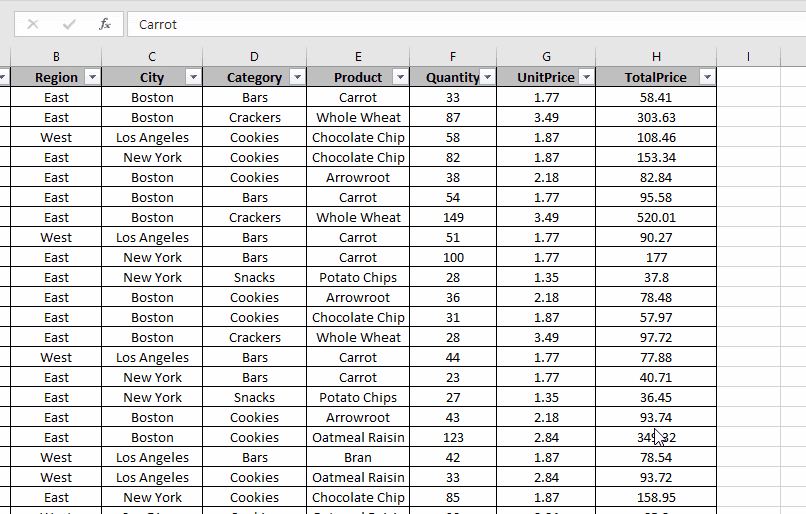As you can see the whole list is filtered with all the products Starting with C.

Note: ? (wildcard) finds single characters.

Tilde (~) wildcard is used when we need to find the symbol question mark or asterisk (not as wildcards). For example if you are searching a string which ends with question mark. You type “ *~?” .This will find you all strings with question mark.

Here we have need to find data ending with *(astrick). So we will apply two wildcards *~* . The first astrick is used as wildcard and the second one is used to find the astrick sign in the data.Note: ~ (wildcard) finds the two characters * &

Not all functions can execute Wildcards with Excel functions.

Here is a list of functions that accepts wildcards.

• AVERAGEIF, AVERAGEIFS
• COUNTIF, COUNTIFS
• SUMIF, SUMIFS
• VLOOKUP, HLOOKUP
• MATCH
• SEARCH

#### Example: Sum the money spent on mango.

In above image, we have this table of amount spend on different fruits in different months. We just need to get total amount spent on mango in all these months.

In I2 the formula is

 =SUMPRODUCT((B2:B9=H2)*C2:E9)

This returns 525 as total amount spent on mangos. You can see this in image above.

##### How does it work?

Well, it is easy. Let’s break down the formula and understand it in pieces.

(B2:B9=H2): This part compares each value in range B2:B9 with H2 and returns an array of TRUE and FALSE. {FALSE;TRUE;FALSE;FALSE;FALSE;FALSE;FALSE;TRUE}.

(B2:B9=H2)*C2:E9: Here we multiplied each value in the above array with values in C2:E9. C2:C9 are also treated as an 2D array. Finally this statement returns a 2D  array of {0,0,0;47,57,67;0,0,0;0,0,0;0,0,0;0,0,0;0,0,0;108,118,128}.

Now SUMPRODUCT looks like this SUMPRODUCT({0,0,0;47,57,67;0,0,0;0,0,0;0,0,0;0,0,0;0,0,0;108,118,128}). It has values of mango only. It sums them up and returns the result as 525.

Another method can be having a totals column and then use it with SUMIF function to get the sum of all columns. But that’s not what we want to do.

SUMIF Function in Excel

As the name suggests, the SUMIF formula in Excel sums values in a range on a given condition.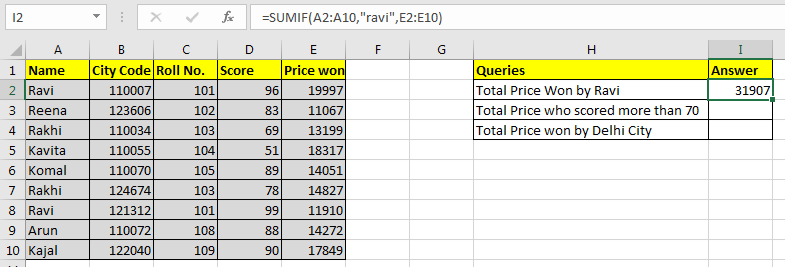Generic Excel SUMIF Formula:

 =SUMIF(condition_range,condition,sum range)

Let's jump into an example. But theory…, Ah! We will cover it later.

#### Use SUMIF To Sum Values On One Condition

For this example I have prepared this data.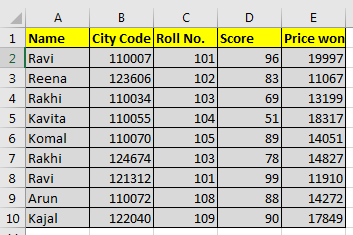Based on this data we need to answer these questions: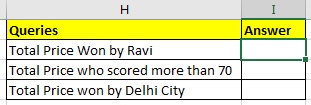#### SUMIF with Text Condition

We need to tell the sum of the price won by Ravi.

So our condition range will be name range and that is A2:A10.

Our condition is Ravi and

Sum range is E2:E10.

So in cell I2 we will write:

 =SUMIF(A2:A10,"ravi",E2:E10)

Note that ravi is in double quotes. Text conditions are always written in double quotes. This is not the case with numbers.

Note that ravi is written in all smalls. Since SUMIF is not case sensitive, hence it doesn’t matter.

The above SUMIF formula will return 31907 as shown in the image below.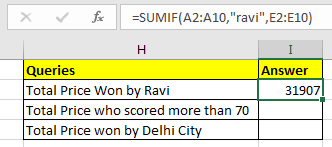#### SUMIF with Logical Operators

For the second question, our condition range will be D2:D10.

Condition is >70 and

The sum range is the same as before.

 =SUMIF(D2:D10,">70",E2:E10)

The above SUMIF formula will return 103973 as shown in the image below.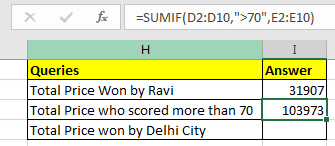##### SUMIF with Wild Card Operators

In the third question, our condition is Delhi. But we don’t have a city column. Hmmm… So what do we have? Aha! City code. This will work.

We know that all Delhi city codes start from 1100. City codes are in 6 digits. So we know it is 1100??. “?” operator is used when we know number of characters but don’t know the character. As here we know that there are two more numbers after 1100. They can be anything, so we used “?”. If didn’t know the number of characters, we would use “*”.

Remember wild card operators only work with text values. Hence you need to convert city code into text.

You can concatenate numbers with “” to make them text value.

 (formula to convert number into text)            = number & “”   or  =CONCATENATE(number,””)

Now in cell I2 write this formula

 =SUMIF(B2:B10,"1100??",E2:E10)

This will return the sum of the price whose city code starts with 1100. In our example it is 79836.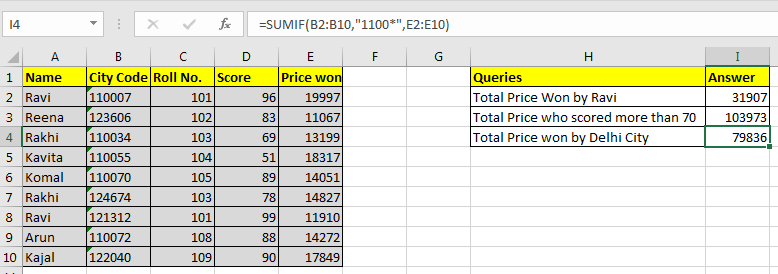Pro Notes:

• If your sum range and condition range are the same, you can omit the sum_range variable in SUMIF function. =SUMIF(E2:E10,">15000") and =SUMIF(E2:E10,">15000",E2:E10) will produce same result, 56163.
• Text values are encapsulated in double quotes, but numbers do not. =SUMIF(C2:C10,103,E2:E10) this will work fine and will return 28026. However while working with logical operators you need to use double quotes. Like our example =SUMIF(D2:D10,">70",E2:E10)
• It can check only one condition. For multiple conditions we use SUMIFS function in Excel.

So yeah guys, this how you can sHow to Use Wildcards?. Let me know if you have any doubts regarding this article or any other article on this site. You can also ask queries regarding Excel 2019, 2016, 2013 and older.

Related Articles

How to use the SUMPRODUCT function in Excel: Returns the SUM after multiplication of values in multiple arrays in excel.

SUM if date is between : Returns the SUM of values between given dates or period in excel.

Sum if date is greater than given date: Returns the SUM of values after the given date or period in excel.

2 Ways to Sum by Month in Excel: Returns the SUM of values within a given specific month in excel.

How to Sum Multiple Columns with Condition: Returns the SUM of values across multiple columns having condition in excel

How to use wildcards in excel : Count cells matching phrases using the wildcards in excel

Popular Articles :

How to use the IF Function in Excel : The IF statement in Excel checks the condition and returns a specific value if the condition is TRUE or returns another specific value if FALSE.

How to use the VLOOKUP Function in Excel : This is one of the most used and popular functions of excel that is used to lookup value from different ranges and sheets.

How to Use SUMIF Function in Excel : This is another dashboard essential function. This helps you sum up values on specific conditions.

How to use the COUNTIF Function in Excel : Count values with conditions using this amazing function. You don't need to filter your data to count specific values. Countif function is essential to prepare your dashboard.

1.